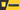### You don't know JavaScript Yet:#3 深入JS的核心## 迭代(Iteration)

### ES6 迭代協定

• done(boolean):若迭代器已迭代完畢整個可迭代序列，則值為`true`。在這個情況下value可以是代表迭代器的回傳值。若迭代器能夠產出序列中的下一個值，則值為`false`。相當於完全不指定`done`屬性。
• value: 任何由迭代器所回傳的值。可於`done``true`時省略。

``````function makeIterator(array) {
let nextIndex = 0;
return {
next() {
const iterator =
nextIndex < array.length
? { value: array[nextIndex], done: false }
: { value: undefined, done: true };
nextIndex += 1;
return iterator;
},
};
}

const it = makeIterator(['a', 'b']);

console.log(it.next()); // { value: "a", done: false }
console.log(it.next()); // { value: "b", done: false }
console.log(it.next()); // { value: undefined, done: true }``````

``````// given an iterator of some data source:
const it = ['a', 'b'];

// loop over its results one at a time
for (const val of it) {
console.log(`Iterator value: \${val}`);
}
//Iterator value: a
//Iterator: b``````

``````class SimpleClass {
constructor(data) {
this.index = 0;
this.data = data;
}

[Symbol.iterator]() {
return {
next: () => {
if (this.index < this.data.length) {
return {value: this.data[this.index++], done: false};
} else {
this.index = 0; //If we would like to iterate over this again without forcing manual update of the index
return {done: true};
}
}
}
};
}

const simple = new SimpleClass([1,2,3,4,5]);

for (const val of simple) {
console.log(val);  //'0' '1' '2' '3' '4' '5'
}``````

`SimpleClass`定義了`[Symbol.iterator]`方法，所以我們可以對它的實例進行迭代。

``````// 將迭代器展開傳遞進陣列中，迭代的value都會儲存於vals當中。
var vals = [ ...it ];

// 將迭代器展開傳遞進函式中，迭代的value會作為參數傳遞。
doSomethingUseful( ...it );``````

`...`的展開形式都遵循迭代器協定(與for..of相同)，以從迭代器中檢索所有可用值並將其放置(展開)到接收上下文中(陣列，或作為參數傳遞)。

``````const greeting = "Hello world!";
const chars = [...greeting]
// [ "H", "e", "l", "l", "o", " ",
//   "w", "o", "r", "l", "d", "!" ]``````

`Map`是一個透過key來獲取值的資料結構，它的迭代方式稍有不同，看看以下的範例:

``````// given two DOM elements, `btn1` and `btn2`

var buttonNames = new Map();
buttonNames.set(btn1,"Button 1");
buttonNames.set(btn2,"Button 2");

for (let [btn,btnName] of buttonNames) {
console.log(`Clicked \${ btnName }`);
});
}``````

``````for (let btnName of buttonNames.values()) {
console.log(btnName);
}
// Button 1
// Button 2``````

``````var arr = [ 10, 20, 30 ];

for (let [idx,val] of arr.entries()) {
console.log(`[\${ idx }]: \${ val }`);
}
// : 10
// : 20
// : 30``````

## 閉包(Closure)

「閉包是讓函式記住與持續訪問在其範疇之外的變數的一種能力，即使該函式在其他範疇中執行也是如此。」

• 所有的函式都是閉包，而物件則不是。
• 若要觀察閉包，我們需要在與最初定義該函式不同範疇之下，執行該函式。

``````function greeting(msg) {
return function who(name) {
console.log(`\${ msg }, \${ name }!`);
};
}

var hello = greeting("Hello");
var howdy = greeting("Howdy");

hello("Kyle");
// Hello, Kyle!

hello("Sarah");
// Hello, Sarah!

howdy("Grant");
// Howdy, Grant!``````

`greeting(..)`會回傳函式`who(..)`的一個實例，`who(..)`中有使用了`greeting(..)`的參數`msg`，當我們第一次執行`greeting(..)`後，將會把參數`msg`的reference分配給`hello`變數，第二次呼叫同理。

`greeting(..)`呼叫完畢後我們通常希望垃圾回收機制能幫我們把所有變數從memory中清除掉，但在上面的例子中`msg`並沒有被清掉，這就是閉包的功能。此時在`hello``howdy`中的`msg`與當初賦予它們的`msg`具有相同的reference，也就是`greeting(..)`範疇的reference，所以實際上這些變數是直接被保留下來的。

``````function counter(step = 1) {
var count = 0;
return function increaseCount(){
count = count + step;
return count;
};
}

var incBy1 = counter(1);
var incBy3 = counter(3);

incBy1();       // 1
incBy1();       // 2

incBy3();       // 3
incBy3();       // 6
incBy3();       // 9``````

``````for (let [idx,btn] of buttons.entries()) {
console.log(`Clicked on button (\${ idx })!`);
});
}``````

## 關鍵字`this`

``````function classroom(teacher) {
return function study() {
console.log(
`\${ teacher } wants you to study \${ this.topic }`
);
};
}

var assignment = classroom("Kyle");``````

``````assignment();
// Kyle wants you to study undefined  -- Oops :(``````

``````var homework = {
topic: "JS",
assignment: assignment
};

homework.assignment();
// Kyle wants you to study JS``````

``````var otherHomework = {
topic: "Math"
};

assignment.call(otherHomework);
// Kyle wants you to study Math``````

## 原型(Prototype)

「原型鏈是將一連串的物件透過prototype連結起來。」

``````var homework = {
topic: "JS"
};``````

`homework`只有`topic`一個屬性，但所有物件預設的prototype都會與物件`Object.prototype`鏈結，其中它有`toString()``valueOf()`等方法， 所以看看下面:

``homework.toString();    // [object Object]``

### 物件鏈結

``````var homework = {
topic: "JS"
};

var otherHomework = Object.create(homework);

otherHomework.topic;
// "JS"``````

`Object.create(..)`的參數允許輸入一個物件，該物件將會與新創建的物件鏈結，然後返回新創建(並鏈結)的物件。看看下面的關係圖就能清楚地看出它們之間的關聯性:

``````homework.topic;
// "JS"

otherHomework.topic;
// "JS"

otherHomework.topic = "Math";
otherHomework.topic;
// "Math"

homework.topic;
// "JS" -- not "Math"``````

### 回頭來看`this`

``````var homework = {
study() {
}
};

var jsHomework = Object.create(homework);
jsHomework.topic = "JS";
jsHomework.study();
`jsHomework``mathHomework`都與`homework`鏈結，`jsHomework.study()`委派給`homework.study()`執行，若在其他語言中，此時的`this`可能只會去尋找`homework`中有沒有`topic`這個屬性， 因為`study()`是定義在`homework`之中，但在JS中它依舊能夠找到`jsHomework`中的`topic`，並且合乎預期的執行，這是JS中`this`的動態能力。